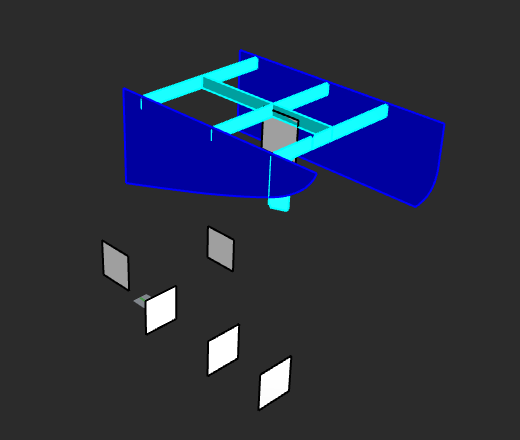# IPlane command implementation using python

Hi All,

Can anyone tell me in python how I can create several Iplanes (infinite plates) from a selection of (planar) surfaces that I can later reference to split my target surfaces?

Hi @g.synetos,

Perhaps this give you a few ideas?

``````import Rhino
import scriptcontext as sc

def _planarsrf_plane(geometry):
face = None
if isinstance(geometry, Rhino.Geometry.Brep) and geometry.IsSurface:
face = geometry.Faces
elif isinstance(geometry, Rhino.Geometry.BrepFace):
face = geometry
if face:
rc, plane = face.TryGetPlane()
if rc:
return plane
return None

def _planarsrf_filter(rhObject, geometry, ci):
plane = _planarsrf_plane(geometry)
if plane:
return True
return False

def test_sel_planarsrf():
go = Rhino.Input.Custom.GetObject()
go.SetCommandPrompt("Select planar surfaces")
go.SetCustomGeometryFilter(_planarsrf_filter)
go.GetMultiple(1, 0)
if go.CommandResult() != Rhino.Commands.Result.Success:
return

planes = []
for objref in go.Objects():
geometry = objref.Geometry()
if geometry:
plane = _planarsrf_plane(geometry)
if plane:
planes.append(plane)

for plane in planes:
print(plane)

if __name__ == "__main__":
test_sel_planarsrf()
``````

– Dale

Hi @dale,

Thanks for the scirpt.
For the attached example I had to change the isinstance check from and to or.
Also i bypassed the _planarsrf_filter as it was popping an exception error.Any suggestion on how to make the created panels to be inside the bounding box of the visible geometry similar to the IPlane command?

The modified script below

``````import Rhino
import scriptcontext as sc
import rhinoscriptsyntax as rs

def _planarsrf_plane(geometry):
face = None
if isinstance(geometry, Rhino.Geometry.Brep) or geometry.IsSurface:
face = geometry.Faces
elif isinstance(geometry, Rhino.Geometry.BrepFace):
face = geometry
if face:
face = geometry.Faces
rc, plane = face.TryGetPlane()
if rc:
return plane
return None

def _planarsrf_filter(rhObject, geometry, ci):
plane = _planarsrf_plane(geometry)
if plane:
return True
return False

def test_sel_planarsrf():
go = Rhino.Input.Custom.GetObject()
go.SetCommandPrompt("Select planar surfaces")
#    go.SetCustomGeometryFilter(_planarsrf_filter)
go.GetMultiple(1, 0)
if go.CommandResult() != Rhino.Commands.Result.Success:
return

planes = []
for objref in go.Objects():
geometry = objref.Geometry()
if geometry:
plane = _planarsrf_plane(geometry)
if plane:
planes.append(plane)

for plane in planes:
#        print(plane)